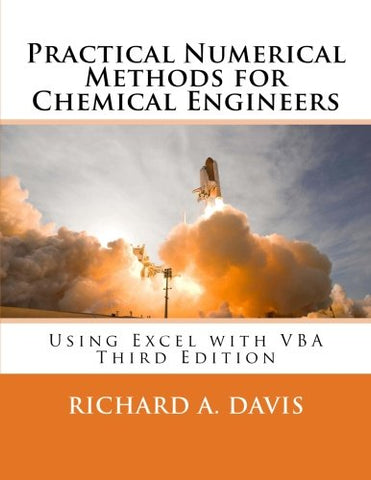# Practical Numerical Methods for Chemical Engineers: Using Excel with VBA, 3rd Edition

• ISBN-13: 9781502527400
• \$194.14

Only 3 left!

This latest edition expands Practical Numerical Methods with more VBA to boost Excel's power for modeling and analysis using the same numerical techniques found in more specialized math software. Visit the companion web site: www.d.umn.edu/~rdavis/PNM/PNMExcelVBA3 to access all of the book's Excel and VBA files, and learn how to customize your own Excel workbooks with: A refined macro-enabled Excel workbook with a suite of over 170 VBA user-defined functions, macros and user-forms for learning VBA and implementing advanced numerical techniques in Excel. More than 200 example and animation workbook files from the book that demonstrate the power of numerical methods. Customize the example files and macros to tackle your own problems using VBA in Excel. Hundreds of practice problems for self-guided study to sharpen your Excel and VBA skills. The first chapter sets the stage for problem solving with numerical methods. The next two chapters cover frequently overlooked features of Excel and VBA for implementing numerical methods in Excel, as well as documenting results. The remaining chapters present powerful numerical techniques using Excel and VBA to find roots to algebraic equations, approximate derivatives, optimize, model data by least-squares regression and interpolation, analyze risk and uncertainty, solve integrals & ordinary & partial differential equations: Numerical Methods & Mathematical Modeling: expert problem solving, Primer on Chemical Reaction Engineering Excel: Documentation, Graphing, Worksheet Functions, Input Validation and Formatting, What-if Analysis VBA: Editor and objects, Function and Sub Procedures, Data Types, Structured Programming, Arithmetic and Worksheet Functions, Flow Control, Arrays, Communication, Message and Input Boxes, User Forms, Reading/Writing Files, Debugging, Unit Conversions Linear Equations: Matrix Algebra, Gaussian Elimination and Crout Reduction with Pivoting, Thomas, Cholesky, Power, Jacobi, and Interpolation Methods for Eigenvalues and Eigenvectors, Jacobi and Gauss-Seidel Iteration, Relaxation Taylor Series Analysis: Finite Difference Derivative Approximation, Richardson's Extrapolation, Ridder's algorithm, Sensitivity Nonlinear Equations Root Finding: Methods of Bisection, Regula Falsi, Newton, Secant, Pade, Wegstein, Quasi-Newton, Aitkin/Steffensen, Homotopy, Bairstow (for polynomial roots), Goal Seek and Solver Optimization: Solver, Luus-Jaakola, Quadratic, Golden Section, Powell, Downhill Simplex, Firefly, Constraints, Scaling and Sensitivity Uncertainty and Risk Analysis: Bootstrap, Confidence Intervals, Law of Propagation, Monte Carlo Simulations with Latin Hypercube Sampling Least-squares Regression: Linear, Nonlinear, LINEST, Gauss-Newton, Levenberg-Marquardt, Validation and Assessment, Uncertainty Analysis, Weighted Regression Interpolation: Linear, Newton Divided Difference and Lagrange Polynomials, Rational, Bulirsh-Stoer, Pade, Stineman, Cubic, B, Akima and Constrained Hermite Splines, Bivariate Interpolation Integration: Graphical, Trapezoidal, Midpoint and transformation for Improper Integrals, Romberg, Adaptive Simpson and Gauss-Kronrod, Multiple Integrals by Simpson, Kronrod and Monte Carlo Initial-value Problems: Single Step Euler and Backward Euler, Implicit Trapezoidal for Stiffness, Variable Step Runge-Kutta Cash-Karp, Dormand-Prince, Multi-step Adams-Bashforth-Moulton, Differential-Algebraic Systems Boundary-value Problems and Partial Differential Equations: Shooting, Finite Difference, Orthogonal Collocation, Quasilinearization, Method of Lines, Crank-Nicholson Review: Reference Tables of Excel and VBA Functions, User-defined Functions, Macros, User Forms